Next: Phase of a rational Up: PHASE OF A MINIMUM-PHASE Previous: PHASE OF A MINIMUM-PHASE

## Phase of a single root

For real, a plot of real and imaginary parts of Z is the circle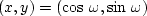.A smaller circle is .9Z. A right-shifted circle is 1+.9Z. Let Z0 be a complex number, such as x0+iy0, or,where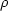and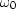are fixed constants. Consider the complex Z plane for the two-term filter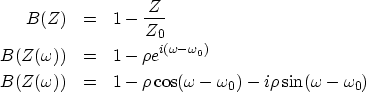(34) (35) (36)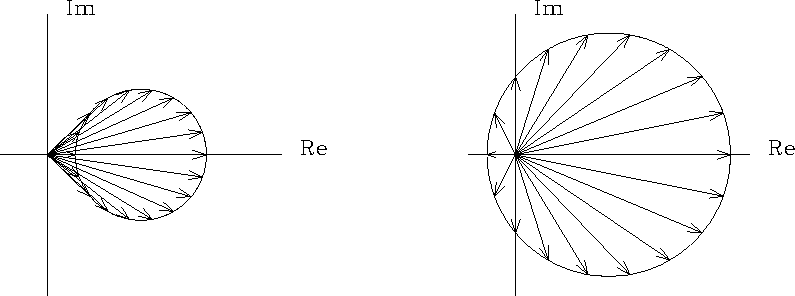origin
Figure 18
Left, complex B plane for. Right, for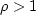.

Real and imaginary parts of B are plotted in Figure 18. Arrows are at frequencyintervals of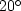.Observe that forthe sequence of arrows has a sequence of angles that ranges over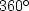,whereas forthe sequence of arrows has a sequence of angles between.Now let us replot equation (37) in a more conventional way, withas the horizontal axis. Whereas the phase is the angle of an arrow in Figure 18, in Figure 19 it is the arctangent of. Notice how different is the phase curve in Figure 19 forthan for.

Real and imaginary parts of B are periodic functions of the frequency,since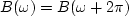.We might be tempted to conclude that the phase would be periodic too. Figure 19 shows, however, that for a nonminimum-phase filter, asranges fromto,the phase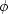increases by(because the circular path in Figure 18 surrounds the origin). To make Figure 19 I used the Fortran arctangent function that takes two arguments, x, and y. It returns an angle betweenand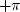.As I was plotting the nonminimum phase, the phase suddenly jumped discontinuously from a value nearto, and I needed to addto keep the curve continuous. This is called phase unwinding.''

 phase Figure 19 Left shows real and imaginary parts and phase angle of equation ((37)), for. Right, for. Left is minimum-phase and right is nonminimum-phase.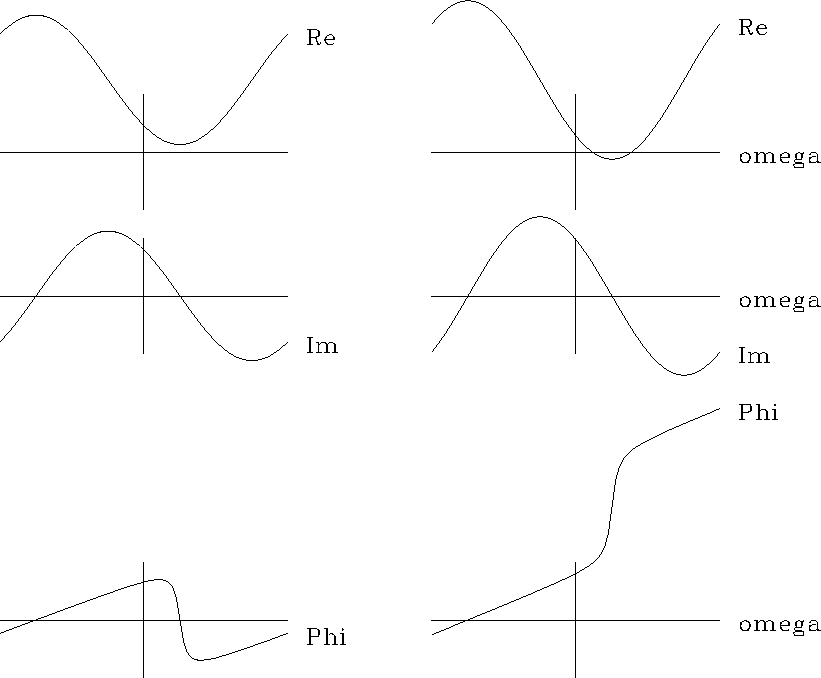You would use phase unwinding if you ever had to solve the following problem: given an earthquake at location (x,y), did it occur in country X? You would circumnavigate the country--compare the circle in Figure 18--and see if the phase angle from the earthquake to the country's boundary accumulated to (yes) or to(no).

The word minimum" is used in minimum phase" because delaying a filter can always add more phase. For example, multiplying any polynomial by Z delays it and addsto its phase.

For the minimum-phase filter, the group delay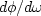applied to Figure 19 is a periodic function of.For the nonminimum-phase filter, group delay happens to be a monotonically increasing function of.Since it is not an all-pass filter, the monotonicity is accidental.

Because group delayis the Fourier dual to instantaneous frequency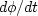,we can now go back to Figure 5 and explain the discontinuous behavior of instantaneous frequency where the signal amplitude is near zero.Next: Phase of a rational Up: PHASE OF A MINIMUM-PHASE Previous: PHASE OF A MINIMUM-PHASE
Stanford Exploration Project
10/21/1998